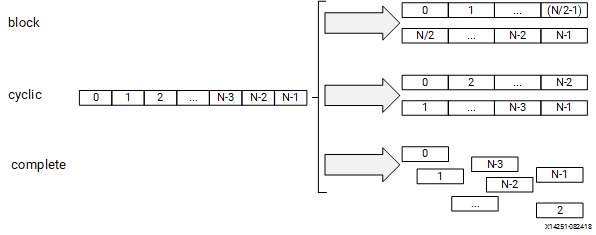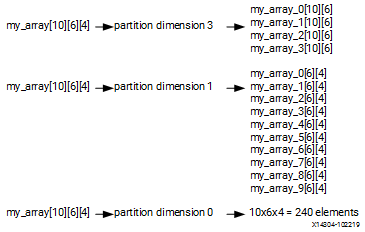# Array Configuration - 2022.1 English

## Vitis Unified Software Platform Documentation: Application Acceleration Development (UG1393)

Document ID
UG1393
Release Date
2022-05-25
Version
2022.1 English

The Vitis compiler maps large arrays to the block RAM memory in the PL region. These block RAM can have a maximum of two access points or ports. This can limit the performance of the application as all the elements of an array cannot be accessed in parallel when implemented in hardware.

Depending on the performance requirements, you might need to access some or all of the elements of an array in the same clock cycle. To achieve this, the can be used to instruct the compiler to split the elements of an array and map it to smaller arrays, or to individual registers. The compiler provides three types of array partitioning, as shown in the following figure. The three types of partitioning are:

• `block`: The original array is split into equally sized blocks of consecutive elements of the original array.
• `cyclic`: The original array is split into equally sized blocks interleaving the elements of the original array.
• `complete`: Split the array into its individual elements. This corresponds to resolving a memory into individual registers. This is the default for the ARRAY_PARTITION pragma.
Figure 1. Partitioning ArraysFor block and cyclic partitioning, the `factor` option specifies the number of arrays that are created. In the preceding figure, a factor of 2 is used to split the array into two smaller arrays. If the number of elements in the array is not an integer multiple of the factor, the later arrays will have fewer elements.

When partitioning multi-dimensional arrays, the `dimension` option is used to specify which dimension is partitioned. The following figure shows how the `dimension` option is used to partition the following example code in three different ways:
``````void foo (...) {
// my_array[dim=1][dim=2][dim=3]
// The following three pragma results are shown in the figure below
// #pragma HLS ARRAY_PARTITION variable=my_array dim=3 <block|cyclic> factor=2
// #pragma HLS ARRAY_PARTITION variable=my_array dim=1 <block|cyclic> factor=2
// #pragma HLS ARRAY_PARTITION variable=my_array dim=0 complete
int  my_array;
...
}``````
Figure 2. Partitioning the Dimensions of an ArrayThe examples in the figure demonstrate how partitioning dimension 3 results in four separate arrays and partitioning dimension 1 results in 10 separate arrays. If 0 is specified as the dimension, all dimensions are partitioned.

## The Importance of Careful Partitioning

A complete partition of the array maps all the array elements to the individual registers. This helps in improving the kernel performance because all of these registers can be accessed concurrently in a same cycle.

CAUTION:
Complete partitioning of the large arrays consumes a lot of PL region. It could even cause the compilation process to slow down and face capacity issue. Partition the array only when it is needed. Consider selectively partitioning a particular dimension or performing a block or cycle partitioning.

## Choosing a Specific Dimension to Partition

Suppose A and B are two-dimensional arrays representing two matrices. Consider the following Matrix Multiplication algorithm:
``````int A;
int B;

ROW_WISE: for (int i = 0; i < 64; i++) {
COL_WISE : for (int j = 0; j < 64; j++) {
#pragma HLS PIPELINE
int result = 0;
COMPUTE_LOOP: for (int k = 0; k < 64; k++) {
result += A[i ][ k] * B[k ][ j];
}
C[i][ j] = result;
}
}``````
Due to the PIPELINE pragma, the `ROW_WISE` and `COL_WISE` loop is flattened together and `COMPUTE_LOOP` is fully unrolled. To concurrently execute each iteration (k) of the `COMPUTE_LOOP`, the code must access each column of matrix A and each row of matrix B in parallel. Therefore, the matrix A should be split in the second dimension, and matrix B should be split in the first dimension.
``````#pragma HLS ARRAY_PARTITION variable=A dim=2 complete
#pragma HLS ARRAY_PARTITION variable=B dim=1 complete
``````

## Choosing between Cyclic and Block Partitions

Here the same matrix multiplication algorithm is used to demonstrate choosing between cyclic and block partitioning and determining the appropriate factor, by understanding the array access pattern of the underlying algorithm.

``````int A[64 * 64];
int B[64 * 64];
#pragma HLS ARRAY_PARTITION variable=A dim=1 cyclic factor=64
#pragma HLS ARRAY_PARTITION variable=B dim=1 block factor=64

ROW_WISE: for (int i = 0; i < 64; i++) {
COL_WISE : for (int j = 0; j < 64; j++) {
#pragma HLS PIPELINE
int result = 0;
COMPUTE_LOOP: for (int k = 0; k < 64; k++) {
result += A[i * 64 +  k] * B[k * 64 + j];
}
C[i* 64 + j] = result;
}
}``````

In this version of the code, A and B are now one-dimensional arrays. To access each column of matrix A and each row of matrix B in parallel, cyclic and block partitions are used as shown in the above example. To access each column of matrix A in parallel, `cyclic` partitioning is applied with the `factor` specified as the row size, in this case 64. Similarly, to access each row of matrix B in parallel, `block` partitioning is applied with the `factor` specified as the column size, or 64.

## Minimizing Array Accesses with Caching

As arrays are mapped to block RAM with limited number of access ports, repeated array accesses can limit the performance of the accelerator. You should have a good understanding of the array access pattern of the algorithm, and limit the array accesses by locally caching the data to improve the performance of the kernel.

The following code example shows a case in which accesses to an array can limit performance in the final implementation. In this example, there are three accesses to the array `mem[N]` to create a summed result.
``````#include "array_mem_bottleneck.h"
dout_t array_mem_bottleneck(din_t mem[N]) {
dout_t sum=0;
int i;
SUM_LOOP:for(i=2;i<N;++i)
sum += mem[i] + mem[i-1] + mem[i-2];
return sum;
}``````
The code in the preceding example can be rewritten as shown in the following example to allow the code to be pipelined with a II = 1. By performing pre-reads and manually pipelining the data accesses, there is only one array read specified inside each iteration of the loop. This ensures that only a single-port block RAM is needed to achieve the performance.
``````#include "array_mem_perform.h"
dout_t array_mem_perform(din_t mem[N]) {
din_t tmp0, tmp1, tmp2;
dout_t sum=0;
int i;
tmp0 = mem;
tmp1 = mem;
SUM_LOOP:for (i = 2; i < N; i++) {
tmp2 = mem[i];
sum += tmp2 + tmp1 + tmp0;
tmp0 = tmp1;
tmp1 = tmp2;
}
return sum;
}``````